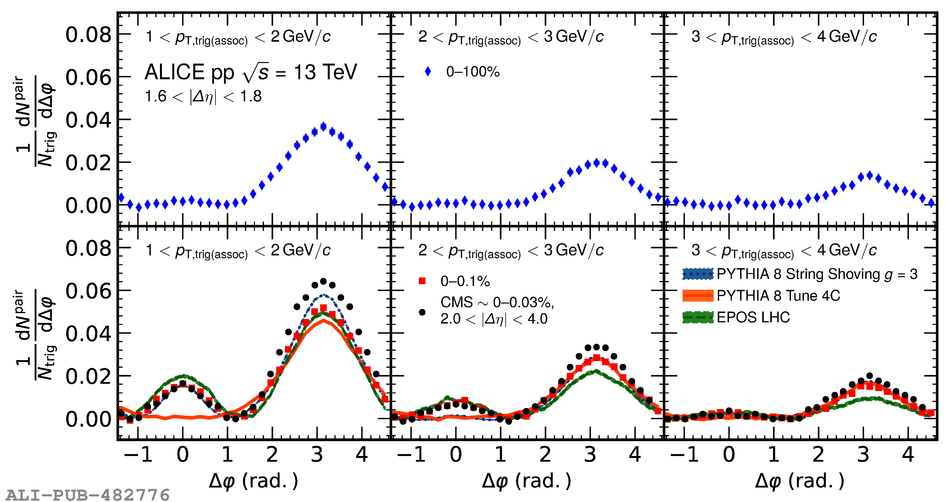# Figure 2

 < p>One-dimensional $\Delta\varphi$ distribution in the large $\Delta\eta$ projection for three transverse momentum intervals in minimum bias (upper panels) and high-multiplicity (lower panels) events after ZYAM subtraction. Transverse momentum intervals of the trigger particles and associated particles are 1~\$ < /p>